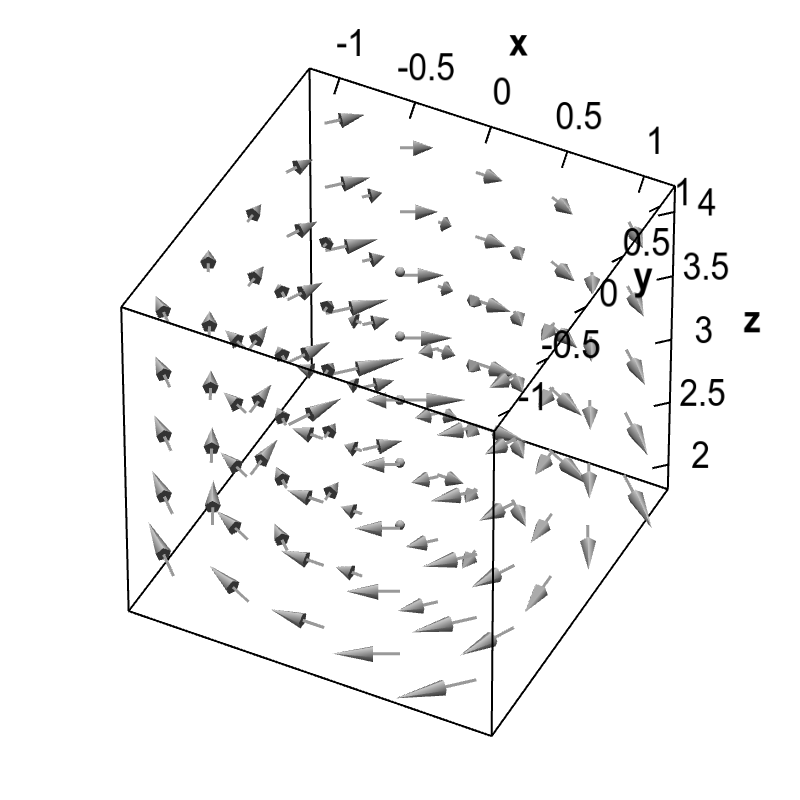# Math Insight

### Vector fields as fluid flow

As described in the vector field overview, a two-dimensional vector field is a vector-valued function $\dlvf:\R^2 \to \R^2$ (confused?) that one can visualize with a field of arrows. For example, the below graph is a visualization of the vector field $\dlvf(x,y)=(y,-x)$.

One can think of such a vector field as representing fluid flow in two dimensions, so that $\dlvf(x,y)$ gives the velocity of a fluid at the point $(x,y)$. In this case, we may call $\dlvf(x,y)$ the velocity field of the fluid. With this interpretation, the above example illustrates the clockwise circulation of fluid around the origin.

The same interpretation is possible for a three-dimensional fluid flow with velocity represented by a vector field $\dlvf:\R^3 \to \R^3$. In this case, $\dlvf(x,y,z)$ is the velocity of the fluid at the point $(x,y,z)$, and we can visualize it as the vector $\dlvf(x,y,z)$ positioned a the point $(x,y,z)$. For example, $\dlvf(x,y,z)=(y/z,-x/z,0)$ can be viewed as fluid circulating around the $z$-axis.